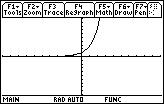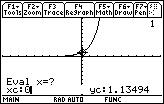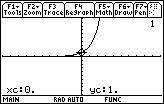# Knowledge Base

## Solution 12141: Graphing and Evaluating Exponential Functions Using the TI-89 Family, TI-92 Family, and Voyage™ 200 Graphing Calculators.

### How can I graph and evaluate an exponential function using a TI-89 family, TI-92 family, or Voyage 200 graphing calculator?

To graph an exponential function, refer to the steps below:

• Press [◊] [Y=] to go to the Y= editor
• Press [2nd] and [ex]
• Press [X] then press [)] to close the parentheses
• Press [ENTER] (screen should read y1= ex)
• Press [◊] [GRAPH] to display the exponential curveWhile on the graph screen, you can then evaluate the exponential function at any value of x.  This can be used to find the y-intercept of the function by evaluating it at x=0.  The following demonstrate how this is done on the calculator:

• Press [F5]  to select 1:Value from the Math menu• Input the desired value of x (0 in this example)
• Press [ENTER] to display the corresponding y-coordinatePlease see the TI-89 family, TI-92 family and Voyage 200 guidebooks for additional information.